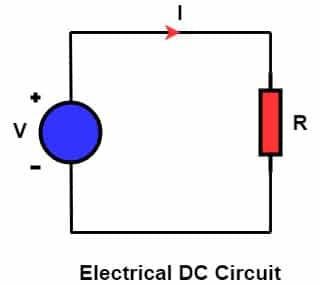# What is Electric Power? Definition, Unit, Types, Formula, Solved Problems

## Electrical Power Definition

The rate at which work is done is called an electrical power. An electrical source has electrical charges stored in it. Therefore, the rate of transfer of electrical charges is electrical power. Thus, the more the transfer rate of the charges, the more the power of the source. In other words, the rate of transfer of electrical charge converts electrical energy into other forms of energy. Unit of electrical power is the watt.

For example, a heater receives electrical energy, and it converts it into heat energy. The AC generator or alternator generates the electrical power. The power is then transferred to a distant location for its utilization.

### Electrical Power Formula

The formula of electrical power is given by

 P = Work done/ Time = Energy/ Time = VIt/t = VI
 P= VI

Where,

P = Power
V = Potential Difference in the circuit
I  =  Current in the circuit

We know, according to Ohm’s law, V= IR

Where,

V= Potential difference in the circuit
I= Circuit current
R = Resistance in the circuit

The other form of electrical power formulas are given by

 P = I2R P = V2/R

### Units of Electrical Power

Unit of electrical power is the watt.

If a potential difference of 1 volt is across an electrical circuit, and the circuit draws 1-ampere current, then the power consumed by the circuit is 1 watt.

P = VI

If,     V = 1 Volt, I = 1 Ampere,   P= 1 Watt

The bigger unit of power is Kilowatt(kW), Gigawatt (GW), and Megawatt(MW).

## Types of Electrical Power

There are two types of electrical power

• DC Power
• AC Power

## 1. DC Power

The current flows in the same direction with the applied DC voltage. Therefore, power is equal to the product of voltage and current. The DC generator, fuel cell, and battery produce DC power.P = VI

Where,

P = Power in Watt
V= Voltage in Volts
I = Current in amperes

## 2.AC Power

The current in the AC circuit does not remain in the phase with the voltage. Yes, the current and voltage in the AC circuit are in phase for the pure resistive circuit. We classify AC power in three categories. These are active power, reactive power, and apparent power.

#### 1.Active Power

The active power (P) is the real power consumed by an electrical circuit. The current in the circuit is in phase with the voltage. The active power in the ac circuit is given by

P = Vrms Irms CosΦ

Where,

P – the real power in watts.
Vrms – RMS voltage
Irms – RMS current
Φ – Phase angle between voltage and current.

#### 2.Reactive Power

Reactive power (Q) is the watt-less power. We measure reactive power in volt-ampere reactive. The circuit draws a reactive current if the load is inductive or capacitive type. The current is out of phase with the applied voltage. The reactive power in the ac circuit is given by

Q = Vrms Irms SinΦ

Where,

P      – the real power in watts.
Vrms – RMS voltage
Irms – RMS current
Φ     – Phase angle between voltage and current.

#### 3.Apparent Power

Apparent power (S) is the vector sum of the active and reactive power. The relationship between active, reactive, and apparent power is given below.### Solved Problems on Electrical Power

Q. 1 A 220 Volts heater draws 4-ampere current from the power source. What is the power consumed by the heater?

P= VI = 220 x 4 = 880 Watts

Q. 2 Calculate the power of an electrical circuit consisting of resistance 4 Ω and a circuit current is 5 A?

R = 4 Ω
I = 5 A

P = I2R = 5 x 5 x 4 = 100 Watts

Q. 3 Calculate the power of an electrical circuit consisting of resistance 4 Ω and voltage applied is 220 volts.

R = 4 Ω
I = 220 A

P = V2/R = 220 x 220 / 4 = 12.1 kW